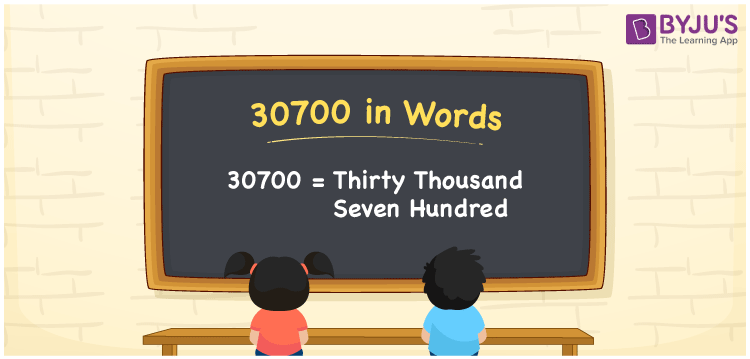# 30700 in Words

30700 in words can be written as Thirty Thousand Seven Hundred. The fundamental concepts in Mathematics like counting or count can be learnt efficiently here. If you buy a washing machine for Rs. 30700, then you can say that “I bought a washing machine for Thirty Thousand Seven Hundred Rupees”. To write numbers in words, the English alphabet is used. The numbers in words concept is explained here in a simple way to improve the conceptual knowledge of students. The number 30700 can be read as “Thirty Thousand Seven Hundred” in English.

 30700 in words Thirty Thousand Seven Hundred Thirty Thousand Seven Hundred in Numbers 30700

## 30700 in English Words## How to Write 30700 in Words?

Students can learn about the expanded form and the place value chart of 30700. Five digits are present in the number 30700. With the help of the place value chart given below, students will be able to understand the concepts with ease.

 Ten Thousands Thousands Hundreds Tens Ones 3 0 7 0 0

30700 can be written in expanded form as:

3 x Ten Thousand + 0 x Thousand + 7 × Hundred + 0 × Ten + 0 × One

= 3 x 10000 + 0 x 1000 + 7 × 100 + 0 × 10 + 0 × 1

= 30000 + 700

= 30700

= Thirty Thousand Seven Hundred

Hence, 30700 in words is written as Thirty Thousand Seven Hundred.

30700 is a natural number that precedes 30701 and succeeds 30699.

30700 in words – Thirty Thousand Seven Hundred

Is 30700 an odd number? – No

Is 30700 an even number? – Yes

Is 30700 a perfect square number? – No

Is 30700 a perfect cube number? – No

Is 30700 a prime number? – No

Is 30700 a composite number? – Yes

## Frequently Asked Questions on 30700 in Words

### How to write 30700 in words?

30700 can be written in words as “Thirty Thousand Seven Hundred”.

### How to write Thirty Thousand Seven Hundred in numbers?

Thirty Thousand Seven Hundred in numbers can be written as 30700.

### Is 30700 an odd or even number?

30700 is an even number as it is completely divisible by 2.